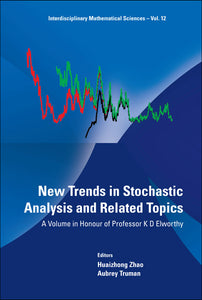# New Trends In Stochastic Analysis And Related Topics: A Volume In Honour Of Professor K D Elworthy

Huaizhong Zhao

Format: Print Book

ISBN: 9789814360913

• SGD 199.02
Unit price per
Tax included.

The volume is dedicated to Professor David Elworthy to celebrate his fundamental contribution and exceptional influence on stochastic analysis and related fields. Stochastic analysis has been profoundly developed as a vital fundamental research area in mathematics in recent decades. It has been discovered to have intrinsic connections with many other areas of mathematics such as partial differential equations, functional analysis, topology, differential geometry, dynamical systems, etc. Mathematicians developed many mathematical tools in stochastic analysis to understand and model random phenomena in physics, biology, finance, fluid, environment science, etc. This volume contains 12 comprehensive review/new articles written by world leading researchers (by invitation) and their collaborators. It covers stochastic analysis on manifolds, rough paths, Dirichlet forms, stochastic partial differential equations, stochastic dynamical systems, infinite dimensional analysis, stochastic flows, quantum stochastic analysis and stochastic Hamilton Jacobi theory. Articles contain cutting edge research methodology, results and ideas in relevant fields. They are of interest to research mathematicians and postgraduate students in stochastic analysis, probability, partial differential equations, dynamical systems, mathematical physics, as well as to physicists, financial mathematicians, engineers, etc.

Format: Hardcover
No of Pages: 460
Imprint: World Scientific
Publication date: 20111129
Series: Interdisciplinary Mathematical Sciences